Study Materials

# NCERT Solutions for Class 9th Mathematics

Page 1 of 3

## Chapter 6. Lines and Angles

### Exercise 6.1

Exercise 6.1

Q1.  In Fig. 6.13, lines AB and CD intersect at O. If  AOC + ∠ BOE = 70° and ∠BOD = 40° find ∠BOE and reflex COE.

Solution: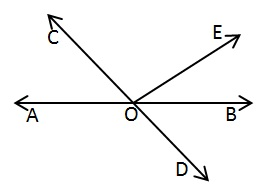∠BOD = 40°

AOC  = ∠BOD (Vertically opposite Angle)

AOC = 40°

AOC  + ∠ BOE = 70° (Given)

BOE = 70°

BOE = 70° - 40°

∠BOE = 30°

AOB is straight line

AOC +  COE +BOE = 180° (linear pair)

⇒ 70° + ∠COE = 180°

∠COE = 180° - 70°

∠COE = 110°

Reflex ∠COE = 360 - 110°

= 250°

Q2. In Fig. 6.14, lines XY and MN intersect at O. If POY = 90° and a : b = 2 : 3, find c.

Solution: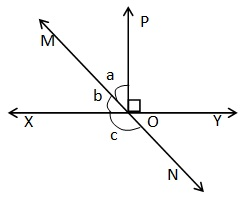POY=90° (given)

Let  a and b = 2x and 3x

XOY is a straight line

a + b + POY = 180°

2x + 3x + 90°= 180°

5x  = 180° ­­- 90°

5x = 90°

x = 90°/5

x = 18°

Now a = 2 x 18°

= 36°

b =3 x 18°

= 54°

MON is a straight line

b + c = 180°(linear pair)

54° + c = 180°

⇒∠c = 180°- 54°

=126°

Q3. In Fig. 6.15, ∠PQR = ∠PRQ, then prove that ∠PQS = ∠PRT

Solution :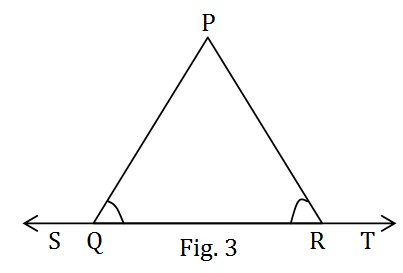Given : ∠PQR = ∠PRQ

To prove : ∠PQS = ∠PRT

Proof :

∠PQS + ∠PQR = 180°  .................. (1)  Linear pair

∠PRT + ∠PRQ = 180°  .................. (2)  Linear pair

From equation (1) and (2)

∠PQS + ∠PQR = ∠PRT + ∠PRQ

Or,  ∠PQS + ∠PQR = ∠PRT + ∠PQR    (∠PQR = ∠PRQ given)Or, ∠PQS = ∠PRT Proved

Q4. In Fig. 6.16, if x + y = w + z, then prove that AOB is a line.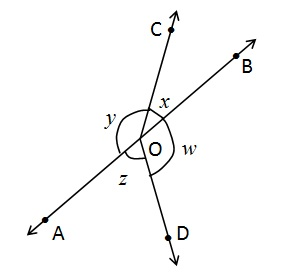Solution:

Given : x + y = w + z

To prove : AOB is a line.

Proof :

We know that;

x + y + w + z = 360

(Angle Sustained on centre)

x + y + x + y =  360 (x + y = w + z given)

2x + 2y = 360

2 (x + y) = 360

x + y = 180 (linear pair)

Therefore, AOB is a line

Hence, Proved

Q5. In Fig. 6.17, POQ is a line. Ray OR is perpendicular to line PQ. OS is another ray lying between rays OP and OR. Prove that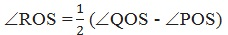Solution:

Given:

POQ is a straight line. OR PQ and OS is

another ray lying between rays OP and OR.

To prove:Proof: OR PQ  (given)

∴ ∠QOR = 90  …………… (1)

POQ is straight line

∴ ∠POR + QOR = 180 (linear pair)

POR + 90 = 180

POR = 180– 90

POR = 90…………… (2)

Now,  ROS + QOR = QOS

Or,       ROS = QOS – QOR  ……………. (3)

Again, ROS + POS = POR

Or,       ROS = POR – POS  ……………. (4)

ROS + ROS = QOS – QOR + POR – POS

2 ROS = QOS – 90+ 90POS

2 ROS = (QOS – POS)Hence Proved

Q6.  It is given that ∠ XYZ = 64° and XY is produced to point P. Draw a figure from the given information. If ray YQ bisects ∠ ZYP, find ∠ XYQ and reflex ∠ QYP.

Solution:Given: ∠ XYZ = 64°and XY is produced to point P. YQ bisects ∠ ZYP.

To Find: ∠XYQ and reflex ∠QYP.

YQ bisects ∠ZYP

∴ ∠ZYQ = ∠QYP   ................. (1)

∵ XY is produced to point P.

∴ PX is a straight line.

Now, ∠ XYZ + ∠ZYQ + ∠QYP = 180° (linear pair)

Or,     64° + ∠ZYQ + ∠QYP = 180°

⇒      ∠ZYQ + ∠QYP = 180° - 64°

⇒      ∠ZYQ + ∠ZYQ = 116°                 [Using equation (1) ]

⇒      2∠ZYQ = 116°

⇒      ∠ZYQ = 116°/2

⇒      ∠ZYQ = 58°

∠ZYQ = ∠QYP = 58°

∠XYQ = ∠XYZ + ∠ZYQ

= 64° + 58°

= 122°

∵ ∠QYP = 58°

∴ Reflex ∠QYP = 360° - 58°

= 302°

∠XYQ  = 122°, Reflex ∠QYP = 302°

Page 1 of 3

Chapter Contents: